# Test: Population Growth

## 8 Questions MCQ Test Practice Questions for GMAT | Test: Population Growth

Description
Attempt Test: Population Growth | 8 questions in 10 minutes | Mock test for GMAT preparation | Free important questions MCQ to study Practice Questions for GMAT for GMAT Exam | Download free PDF with solutions
QUESTION: 1

### Jolene entered an 18-month investment contract that guarantees to pay 2 percent interest at the end of 6 months, another 3 percent interest at the end of 12 months, and 4 percent interest at the end of the 18 month contract. If each interest payment is reinvested in the contract, and Jolene invested \$10,000 initially, what will be the total amount of interest paid during the 18-month contract?

Solution:

The investment contract guarantees to make three interest payments:
\$10,000 (initial investment)
+   \$200 (1% interest on \$10,000 principal
= \$100, so 2% = 2 × \$100) = \$10,200
⇒ \$10,200 +   \$306 (1% interest on \$10,200 principal = \$102, so 3% = 3 × \$102) = \$10,506
⇒ +   \$420.24 (1% interest on \$10,506 principal = \$105.06, so 4% = 4 × \$105.06) = \$10,926.24
The final value is \$10,926.24 after an initial investment of \$10,000. Thus, the total amount of interest paid is \$926.24 (the difference between the final value and the amount invested).

QUESTION: 2

### Wes works at a science lab that conducts experiments on bacteria. The population of the bacteria multiplies at a constant rate, and his job is to notate the population of a certain group of bacteria each hour. At 1 p.m. on a certain day, he noted that the population was 2,000 and then he left the lab. He returned in time to take a reading at 4 p.m., by which point the population had grown to 250,000. Now he has to fill in the missing data for 2 p.m. and 3 p.m. What was the population at 3 p.m.?

Solution:

If we decide to find a constant multiple by the hour, then we can say that the population was multiplied by a certain number three times from 1 p.m. to 4 p.m.: once from 1 to 2 p.m., again from 2 to 3 p.m., and finally from 3 to 4 p.m.

Let's call the constant multiple x.

2,000(x)(x)(x) = 250,000
2,000(x3) = 250,000
x3 = 250,000/2,000 = 125
x = 5

Therefore, the population gets five times bigger each hour.

At 3 p.m., there were 2,000(5)(5) = 50,000 bacteria.

QUESTION: 3

### The population of locusts in a certain swarm doubles every two hours. If 4 hours ago there were 1,000 locusts in the swarm, in approximately how many hours will the swarm population exceed 250,000 locusts?

Solution:

A population problem on the GMAT is best solved with a population chart that illustrates the swarm population at each unit of time. An example of a population chart is shown below: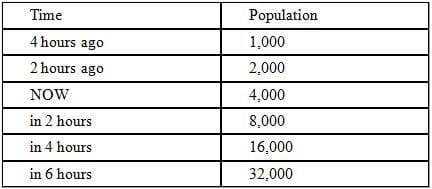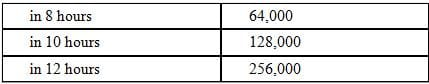As can be seen from the chart, in 12 hours the swarm population will be equal to 256,000 locusts. Thus, we can infer that the number of locusts will exceed 250,000 in slightly less than 12 hours. Since we are asked for an approximate value, 12 hours provides a sufficiently close approximation and is therefore the correct answer.

QUESTION: 4

An investor purchased a share of non-dividend-paying stock for p dollars on Monday. For a certain number of days, the value of the share increased by r percent per day. After this period of constant increase, the value of the share decreased the next day by q dollars and the investor decided to sell the share at the end of that day for v dollars, which was the value of the share at that time. How many working days after the investor bought the share was the share sold, if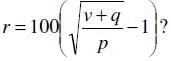Solution:

We need to consider the formula for compound interest for this problem: F = P(1 + r)x, where F  is the final value of the investment, P is the principal, r is the interest rate per compounding period as a decimal, and x is the number of compounding periods (NOTE: sometimes the formula is written in terms of the annual interest rate, the number of compounding periods per year and the number of years). Let's start by manipulating the given expression for r: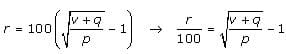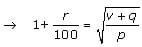→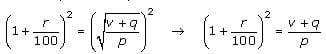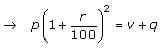→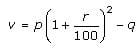Let’s compare this simplified equation to the compound interest formula. Notice that r in this simplified equation (and in the question) is not the same as the r in the compound interest formula. In the formula, the r is already expressed as a decimal equivalent of a percent, in the question the interest is r percent.   The simplified equation, however, deals with this discrepancy by dividing r by 100.
In our simplified equation, the cost of the share of stock (p), corresponds to the principal (P) in the formula, and the final share price (v) corresponds to the final value (F) in the formula.  Notice also that the exponent 2 corresponds to the x in the formula, which is the number of compounding periods. By comparing the simplified equation to the compound interest formula, we see that the equation tells us that the share rose at the daily interest rate of p percent for TWO days. Then the share lost a value of q dollars on the third day, i.e. the “– q” portion of the expression. If the investor bought the share on Monday, she sold it three days later on Thursday.

QUESTION: 5

A certain investment grows at an annual interest rate of 8%, compounded quarterly. Which of the following equations can be solved to find the number of years, x, that it would take for the investment to increase by a factor of 16?

Solution:

Compound interest is computed using the following formula:

F = P ( 1 + r/n)nt, where
F = Final value
P = Principal
r = annual interest rate
n = number of compounding periods per year
t = number of years

From the question, we can deduce the following information about the growth during this period:

At the end of the x years, the final value, F, will be equal to 16 times the principal (the money is growing by a factor of 16).
Therefore,  F = 16P.
r = .08 (8% annual interest rate)
n = 4 (compounded quarterly)
t = x (the question is asking us to express the time in terms of x number of years)

We can write the equation
16P = P (1 + .08/4)4x
16 = (1.02)4x

Now we can take the fourth root of both sides of the equation. (i.e.the equivalent of taking the square root twice) We will only consider the positive root because a negative 2 doesn't make sense here.
161/4 = [(1.02)4x]1/4
2 = (1.02)x

QUESTION: 6

Jim needs \$1,000 to buy a new flat-screen TV. Since he has only \$7, he borrows the remanining balance from his sister Mary. The loan will be repaid in 3 annual installments at an interest rate of 10%, compounded annually. The formula for calculating the monthly payment P is P = (L x C x r) / (C – 1) where L = amount of the loan, r = annual interest rate, and C = compounding factor = (1 + r)N where N = number of annual payments. How much does Jim have to pay Mary at the end of each of the next 3 years (rounded to the nearest penny)?

Solution:

Although this problem appears to be complicated, it is fairly straightforward; since we are given a formula, we can simply plug in the values that we need then calculate. First, let us assign a value to each of the variables in the formula:
L = amount of the loan = 1000 – 7 = 993
r = annual interest rate = 10% = 0.1
C = compounding factor = (1 + r)N = (1.1)3 = (1.1)(1.1)(1.1) = 1.21(1.1) = 1.331
Hence P = (993 x 1.331 x 0.1) / (1.331 – 1) = (993 x 1.331 x 0.1) / 0.331 = (993/.331) x 1.331 x 0.1.
Note that 993 is an integral multiple of 0.331 and 993/0.331 = 993000/331 = 3000.
Hence P = (993/0.331) x 1.331 x 0.1 = 3000 x 1.331 x 0.1 = 399.30

QUESTION: 7

Louie takes out a three-month loan of \$1000. The lender charges him 10% interest per month compounded monthly. The terms of the loan state that Louie must repay the loan in three equal monthly payments. To the nearest dollar, how much does Louie have to pay each month?

Solution:

The question asks us to find the monthly payment on a \$1000 loan at 10% monthly interest compounded monthly for three months. Let's define the following variables:

P = Principal = \$1000
i  = monthly interest rate = 10% = 0.1
c = compound growth rate = 1 + i = 1.1
x = monthly payment (to be calculated)

At the start, Louie's outstanding balance is P. During the next month, the balance grows by a factor of c as it accumulates interest, then decreases by x when Louie makes his monthly payment. Therefore the balance after month 1 is Pc - x. Each month, you must multiply the previous balance by c to accumulate the interest, and then subtract x to account for Louie's monthly payment. In chart form:

Balance at start:          P
Balance after month 1: Pc – x
Balance after month 2: [Pc – x]c – x = Pc2 – x(c+1)
Balance after month 3: [Pc2 - x(c+1)]c - x = Pc3 - x(c2+c+1)

Finally, the loan should be paid off after the third month, so the last loan balance must equal 0. Therefore:

0 = Pc3 - x(c2+c+1)
x(c2+c+1) = Pc3
x = (Pc3) / (c2+c+1)            Note that c = 1.1; c2 = 1.21; c3 = 1.331

x = 1000(1.331) / (1.21+1.1+1)
x = 1331 / 3.31

Rounded to the nearest dollar, x = 402.

QUESTION: 8

Donald plans to invest x dollars in a savings account that pays interest at an annual rate of 8% compounded quarterly. Approximately what amount is the minimum that Donald will need to invest to earn over \$100 in interest within 6 months?

Solution:

The formula for calculating compound interest is A = P(1 + r/n)nt where the variables represent the following:

A = amount of money accumulated after t years (principal + interest)
P = principal investment
r = interest rate (annual)
n = number of times per year interest is compounded
t = number of years

In this case, x represents the unknown principal, r = 8%, n = 4 since the compounding is done quarterly, and t = .5 since the time frame in question is half a year (6 months).

You can solve this problem without using compound interest.  8% interest over half a year, however that interest is compounded, is approximately 4% interest.  So, to compute the principal, it's actually a very simple calculation:

100 = .04x
2500 = xUse Code STAYHOME200 and get INR 200 additional OFF Use Coupon Code